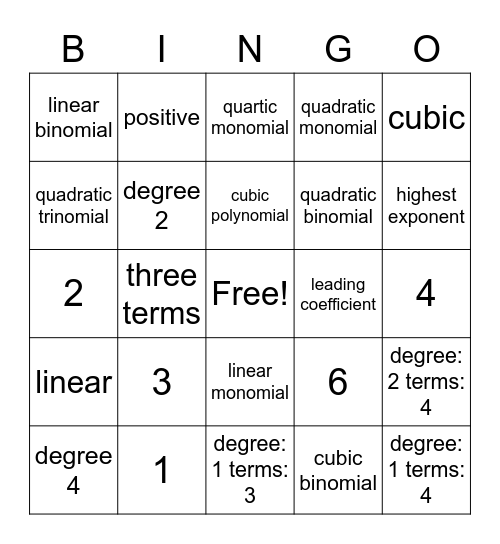# Polynomial Definitions BingoThis bingo card has a free space and 33 words: monomial, binomial, three terms, four or more terms, linear, degree 2, cubic, degree 4, positive, negative, cubic polynomial, quartic monomial, quartic binomial, quartic trinomial, leading coefficient, highest exponent, 1, 2, 3, 6, linear monomial, 5, linear binomial, 4, degree: 1 terms: 3, degree: 1 terms: 4, quadratic monomial, quadratic binomial, quadratic trinomial, degree: 2 terms: 4, cubic monomial, cubic binomial and cubic trinomial.

## Play Online